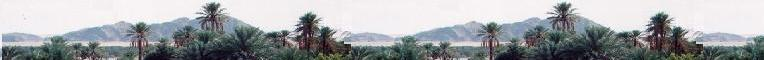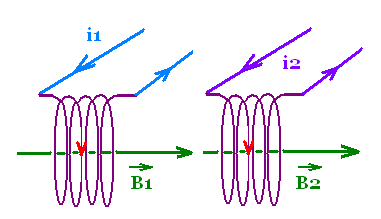Electrostatics

Electromagnetics

Electricity &
Magnetism

# Mutual inductance

### Mutual inductanceConsider two coils 1 and 2 one in front of the other, of length l1 and l2, cross-sectional areas S1 and S2, with n1, and n2 turns per unit length each.

The two coils carry the currents i1 and i2, . The current i1 produces a magnetic field B1 inside the coil 1 that influences the nearby coil 2.

When i1 changes (then B1 changes), it produces in the coil 1 a self-induced emf = ℰ11, and a induced emf = ℰ21 in the coil 2 through (external field) B1.

Similarly, the current i2 produces a magnetic field B2 inside the coil 2 that influences the nearby coil 1.

when i2 changes in the coil 2 (then B2 changes), it produces in the coil 2 a self-induced emf = ℰ22, and a induced emf = ℰ12 in the coil 1 through (external field) B2.

The effect of inducing an emf by one coil on the other is called mutual induction.

Self-induction in the coil 1
The magnetic field inside is B1 = μo n1 i1
The magnetic flux due to B1 that links this coil is Φ11 = B1 S1
Its emf = ℰ1 = - n1 dΦB1/dt
= - μon12 S1 l1 di1/dt
Its self-inductance is L1 = μon12S1l1

Self-induction in the coil 2
The magnetic field inside is B2 = μo n2 i2
The magnetic flux due to B2 that links this coil is Φ22 = B2 S2
Its self = ℰ2 = - n2 dΦB2/dt
= - μon22 S2 l2 di2/dt
Its self-inductance is L12 = μon22S2l2

Interaction: Mutual-induction of the coil 1 and the coil 2

The magnetic field inside the two coils is B1 + B2 = μo n2 i2 + μo n1 i1

The magnetic flux due to (B1 + B2) that links this coil 1 is Φ1-12 = (B1 + B2) S1
and the magnetic flux due to (B1 + B2) that links this coil 2 is Φ2-12 = (B1 + B2) S2

The emf of the coil 1 is emf = ℰ12
= - n1 l1 dΦ(B1 + B2)/dt
= - n1 l1 S1 μo d(n2 i2 + n1 i1)/dt
= - n1 l1 S1 μo (n2 di2/dt + n1 di1/dt)

The emf of the coil 2 is emf = ℰ21
= - n2 l2 dΦ(B1 + B2)/dt
= - n12 l2 S2 μo d(n2 i2 + n1 i1)/dt

We define:

The mutual-inductance for the coil 1:
12 = - M12 di1/dt, and
The mutual-inductance for the coil 2:
21 = - M21 di2/dt, and

M12 and M21 are called coefficient of mutual inductance. They depend on the geometrical factors of the coils.

We can consider the particular case where M12 = M21 = M. M is then the inductance of the pair. The SI unit of the mutual inductance is the Henry.

 chimie labs | Physics and Measurements | Probability & Statistics | Combinatorics - Probability | Chimie | Optics | contact |